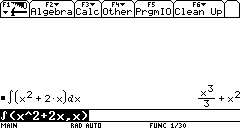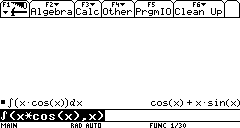# Knowledge Base

## Solution 12114: Calculating Integrals on the TI-89 Titanium and Voyage™ 200 Graphing Calculators.

### How do I calculate integrals on the TI-89 Titanium and Voyage 200 graphing calculators?

The following examples demonstrate how to perform indefinite and definite integrals on the TI-89 Titanium and Voyage 200 graphing calculators:

Indefinite integrals:

Please Note: The ∫ symbol is the 2nd function of the "7" key.

Example 1: To find the integral of x2+2x:

1) On the Home screen, enter ∫(x^2+2x,x).
2) Press [ENTER] to display the answer.Example 2: To find the integral of x*cos(x):

1) On the Home screen, enter ∫(x*cos(x),x).
Please Note: The multiplication sign between x and cos(x) is required.
2) Press [ENTER] to display the answer.Definite integral

Example: To find the integral of x3+x from in the interval [1,3]:

1) On the Home screen, enter ∫(x^3+x,x,1,3).
2) Press [ENTER] to display the answer.Please Note: When using definite integrals to find the area below a graph, first graph the function to see if parts of the area being calculated is not below the x-axis and take it into account when using the integration.

Please see the TI-89 family, TI-92 family and Voyage 200 guidebooks for additional information.